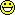## Speed improvement [🇷 for BE/BA]

Hi again,

now it gets really interesting.

1. There is this 30% improvement if I switch to profile log likelihood in stead of "the big one" for lac kof betyter wording
2. I can achieve about an improvement of several thousand percent if stay with the big X and V, however, I must then use a smart tactic:
I am only generating V once, but though each pass on the optimiser I am updating V rather than re-writing it.
Instead of iterating across all rows and column and checking in the data list where to put the individual components, I am initially generating a list of coordinates for each variance component. Something like this:

V.Coordinates=function(Data) {  Nobs=nrow(Data)  xy.varT=NULL; xy.varBR=NULL; xy.varWR=NULL; xy.varBRT=NULL;  for (iRow in 1:Nobs)  for (iCol in 1:Nobs)   {    ## the diagonal    if (iCol==iRow)     {       if (Data$Trt[iRow]=="T") xy.varT=rbind(xy.varT, c(iRow, iCol)) if (Data$Trt[iRow]=="R") xy.varWR=rbind(xy.varWR, c(iRow, iCol))     }    ## off diagonal    if (iCol!=iRow)    if (Data$Subj[iRow]==Data$Subj[iCol])     {      if (Data$Trt[iCol]==Data$Trt[iRow]) xy.varBR=rbind(xy.varBR, c(iRow, iCol))      if (Data$Trt[iCol]!=Data$Trt[iRow]) xy.varBRT=rbind(xy.varBRT, c(iRow, iCol))     }   }  return(list(xy.varT=xy.varT, xy.varBR=xy.varBR, xy.varWR=xy.varWR, xy.varBRT=xy.varBRT)) }

This returns a list with four components. The first component is a set of coordinates in V for varT, the second is a set of coordinates for varBR. And so forth.

Then, in the REML function, I am having a block like this:
   for (i in 1:nrow(VC$xy.varT)) V[VC$xy.varT[i,1], VC$xy.varT[i,2]] = Pars for (i in 1:nrow(VC$xy.varBR))    if (VC$xy.varBR[i,1] != VC$xy.varBR[i,2])     V[VC$xy.varBR[i,1], VC$xy.varBR[i,2]] = Pars   for (i in 1:nrow(VC$xy.varWR)) V[VC$xy.varWR[i,1], VC$xy.varWR[i,2]] = Pars+Pars for (i in 1:nrow(VC$xy.varBRT))    V[VC$xy.varBRT[i,1], VC$xy.varBRT[i,2]] = Pars

And now are simply talking REAL BUSINESSThe optimiser is returning blazingly fast with an REML estimate and corresponding variance components. Depending on the tolerance required it is almost instantaneous (<0.5 secs) on my laptop and that machine isn't a high tech equipment at all. The speed with "big V" far outruns the profile approach.
I believe this implies that matrix inversion is done in a clever fashion in R's solve function; as you pointed out PharmCat inversion can be slow (and unstable) as matrices get large, but perhaps the LAPACK routine used by R reduces the task intelligently, possibly through some of the same tricks described for e.g. the Alglib routines..

However, I am also seeing a different little thing:
If I optimise with the profile approach I can make BFGS converge, but this will not work if I try it it in the "big V" approach. Lindstrom and Bates noticed something similar in the sense that they wrote that Quasi-Newton methods perform better when the profile approach is used:
"We recommend optimizing the profile log- likelihood, since it will usually require fewer iterations, the derivatives are somewhat simpler, and the convergence is more consistent. We have also encountered examples where the NR algorithm failed to converge when optimizing the likelihood including a but was able to optimize the profile likelihood with ease."

So, lots of options and I am not going to be late for supperPass or fail!
ElMaestroIng. Helmut Schütz